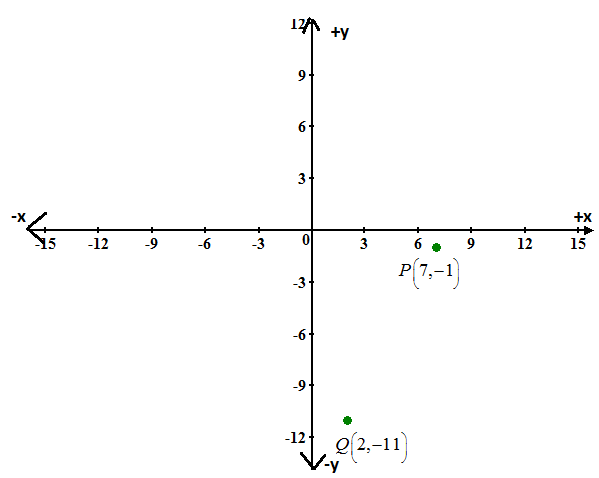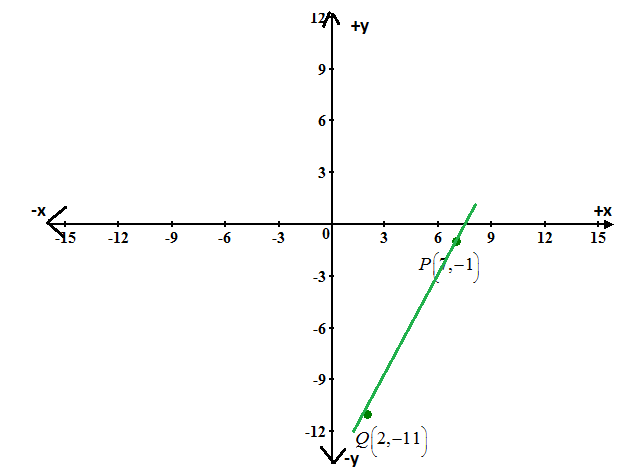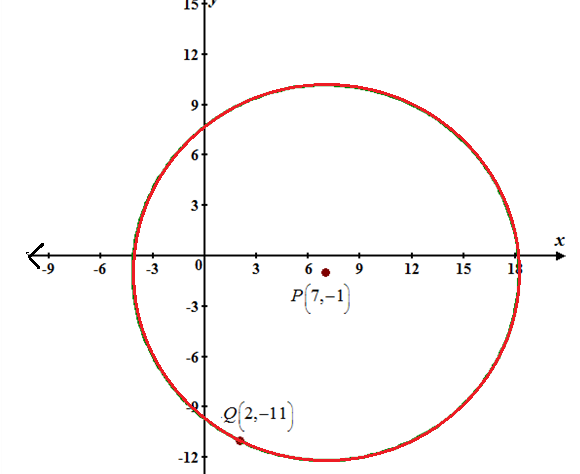# Plot the points on coordinate plane.### Precalculus: Mathematics for Calcu...

6th Edition
Stewart + 5 others
Publisher: Cengage Learning
ISBN: 9780840068071### Precalculus: Mathematics for Calcu...

6th Edition
Stewart + 5 others
Publisher: Cengage Learning
ISBN: 9780840068071

#### Solutions

Chapter 1, Problem 100RE

a.

To determine

## Plot the points on coordinate plane.

Expert Solution### Explanation of Solution

Given information:

The given points are, P(7,1),Q(2,11) .

Calculation:

Consider the given points,

P(7,1),Q(2,11)

Now plot the points on coordinate plane.Hence, the points are plotted.

b.

To determine

Expert Solution

d=125 .

### Explanation of Solution

Given information:

The given points are, P(7,1),Q(2,11) .

Calculation:

Consider the given points,

P(7,1),Q(2,11)

Now apply distance formula,

d=(x2x1)2+(y2y1)2d=(27)2+(11+1)2=(5)2+(10)2=25+100d=125

Hence, the distance between the points is d=125 .

c.

To determine

Expert Solution

92,6 .

### Explanation of Solution

Given information:

The given points are, P(7,1),Q(2,11) .

Calculation:

Consider the given points,

P(7,1),Q(2,11)

Now apply midpoint formula for points (x1,y1),(x2,y2) ,

x=x1+x22,y=y1+y22x=7+22,y=1112x=9/2,y=6

Hence, midpoints are 92,6 .

d.

To determine

Expert Solution

y=(127)x247 .

### Explanation of Solution

Given information:

The given points are, P(7,1),Q(2,11) .

Calculation:

Consider the given points,

P(7,1),Q(2,11)

Line plotted between the points,Now find slope of line to calculate the equation in slope-intercept form.

y=mx+bm=y2y1x2x1=11+127=105m=2

To find yintercept line passes through points (7,1) .

y=2x+b1=2×7+bb=15[y=mx+b]y=2x15

Hence, the equation in slope-intercept form is, y=2x15

e.

To determine

### Find the equation of this circle and draw the circle.

Expert Solution

(x7)2+(y+1)2=125### Explanation of Solution

Given information:

The given points are, P(7,1),Q(2,11) .

Calculation:

Consider the given points,

P(7,1),Q(2,11)

The standard formula for circle has radius r centred at (a,b) centred point (7,1) circle passes through the points (2,11) is,

Radius equal to distance between two points, r=125

(xa)2+(yb)2=r2r=125a=7,b=1now,putthevalues,(x7)2+(y+1)2=125Hence, the equation of circle is (x7)2+(y+1)2=125 .

### Have a homework question?

Subscribe to bartleby learn! Ask subject matter experts 30 homework questions each month. Plus, you’ll have access to millions of step-by-step textbook answers!